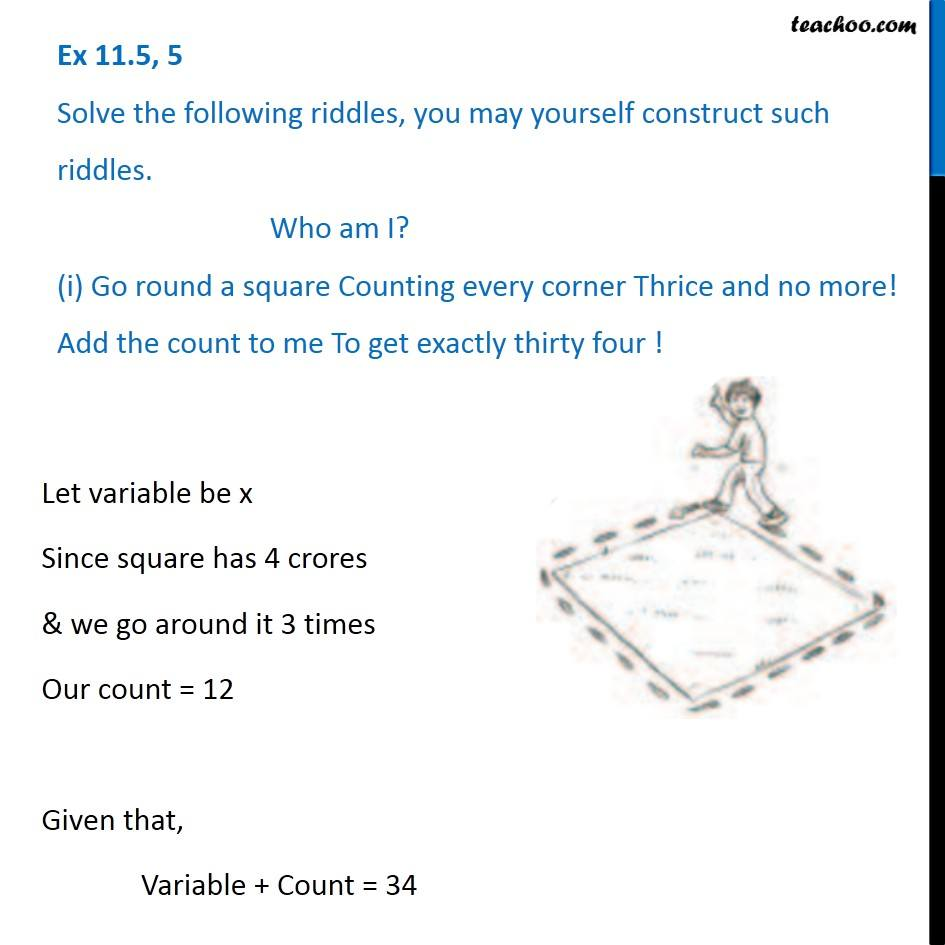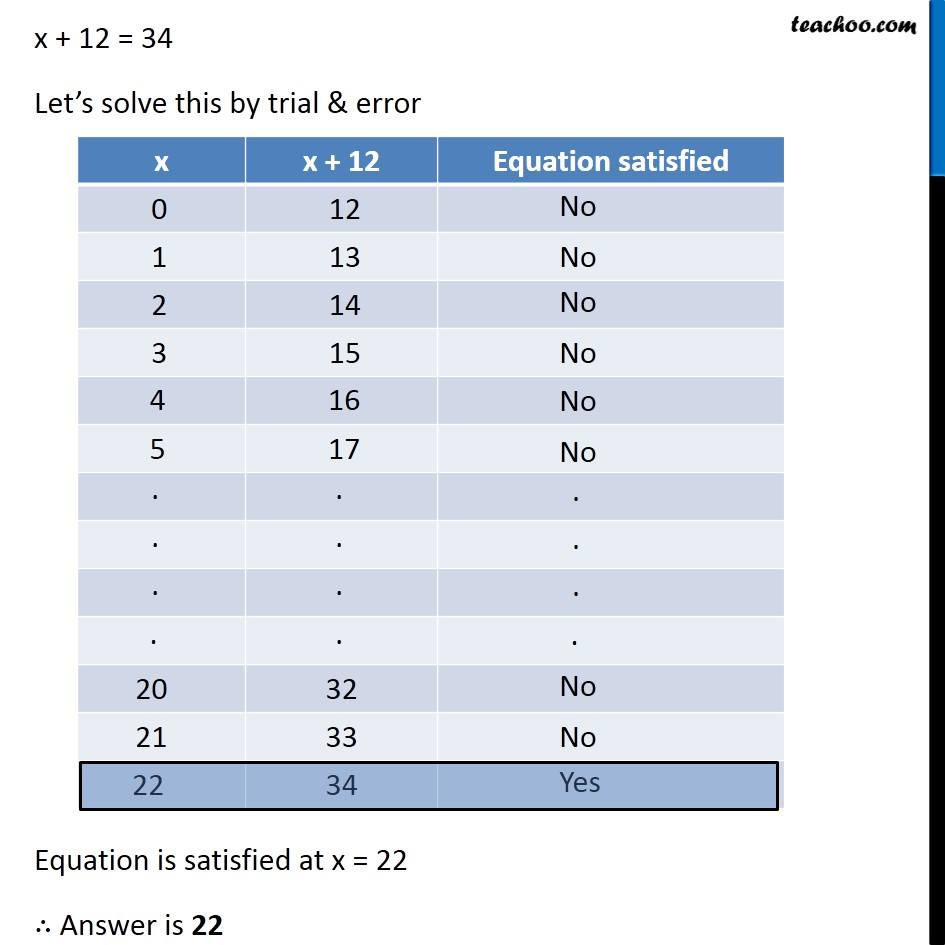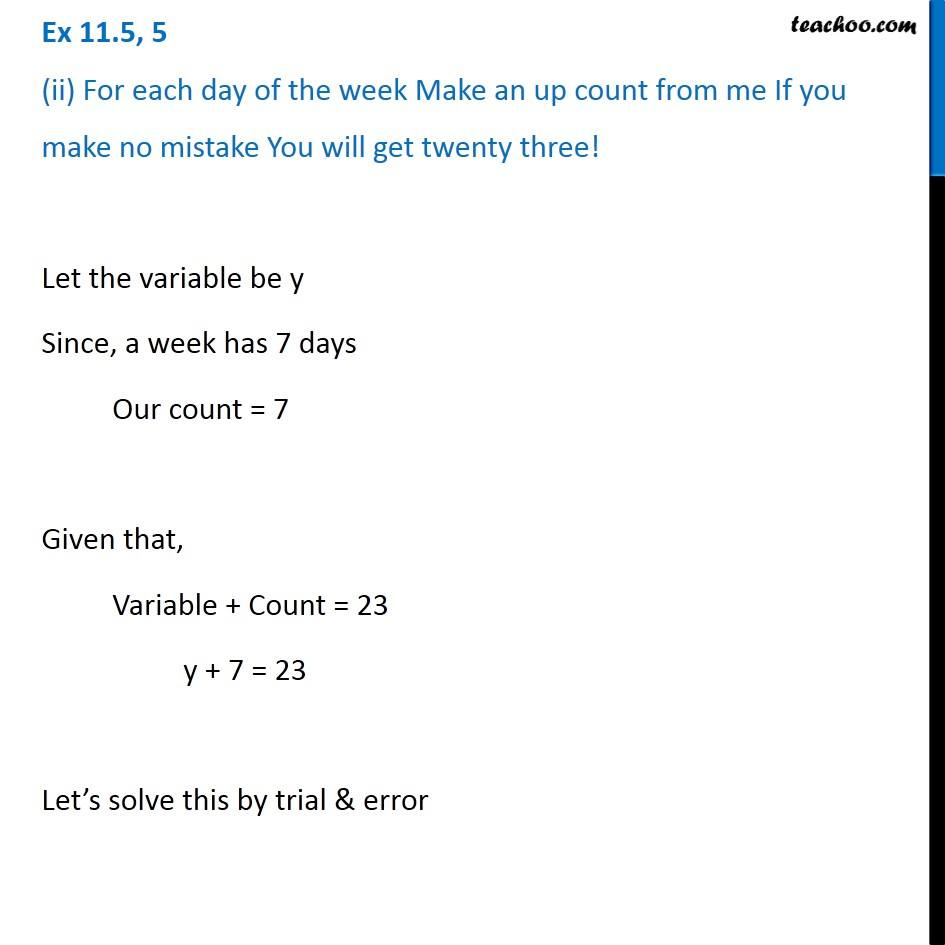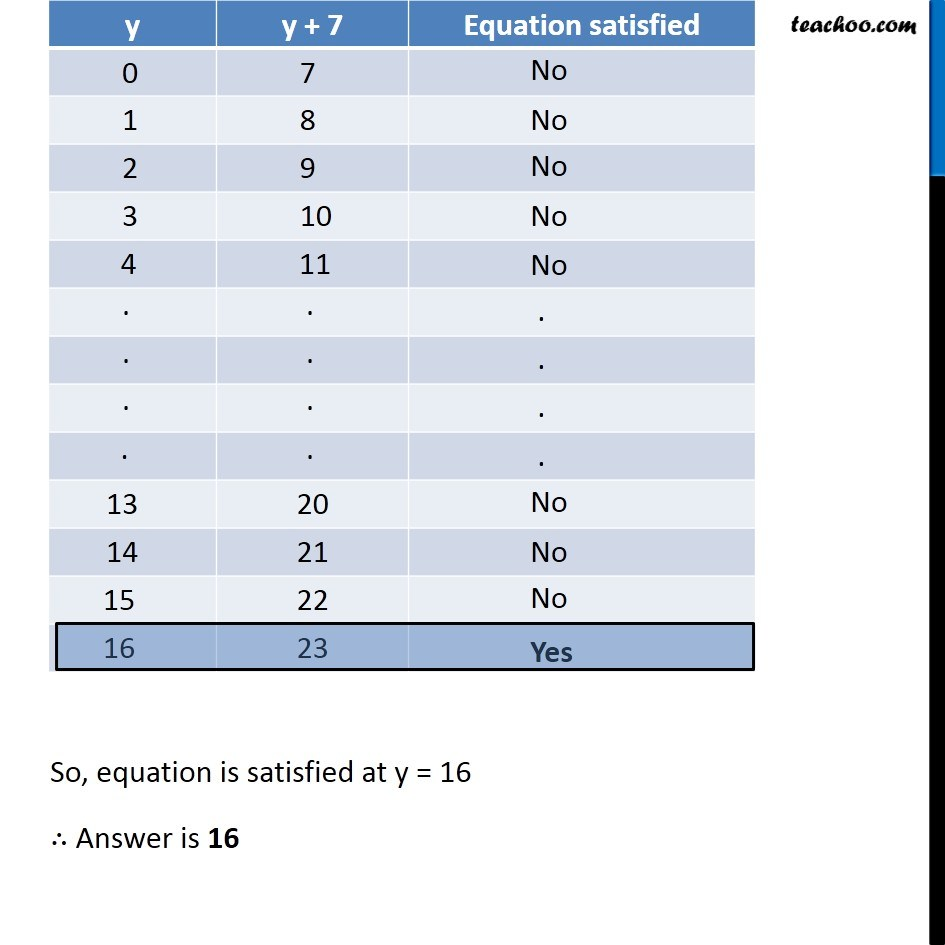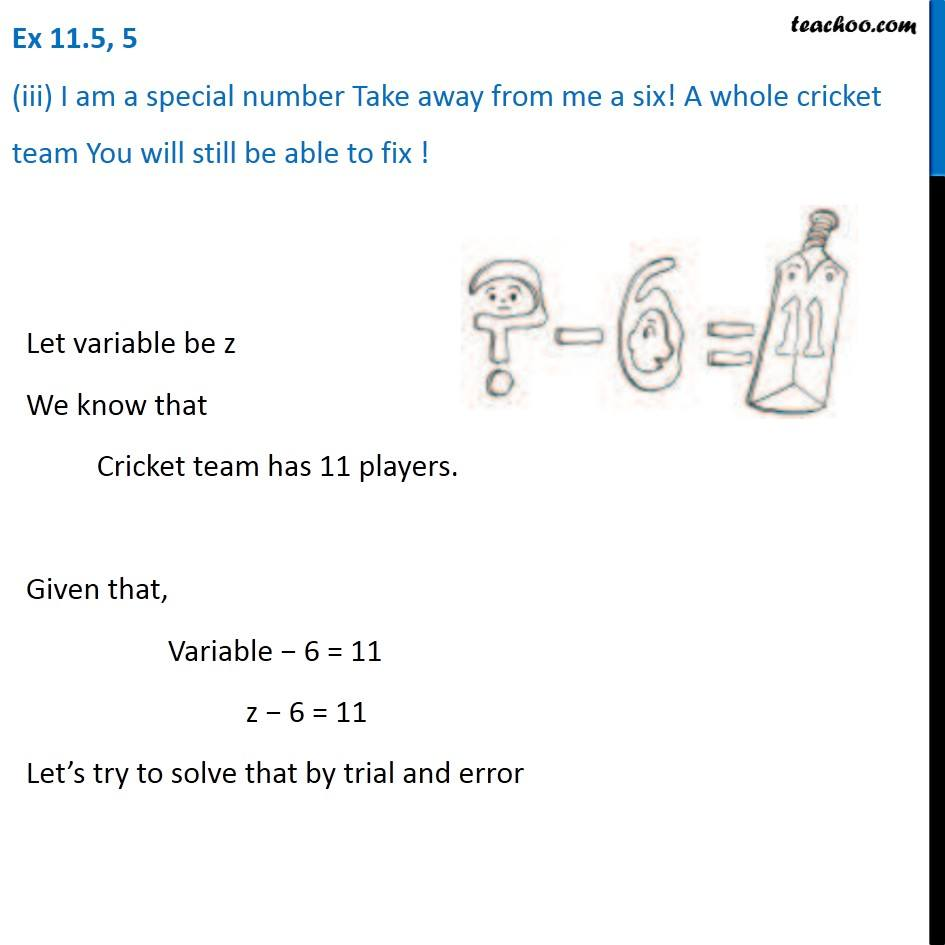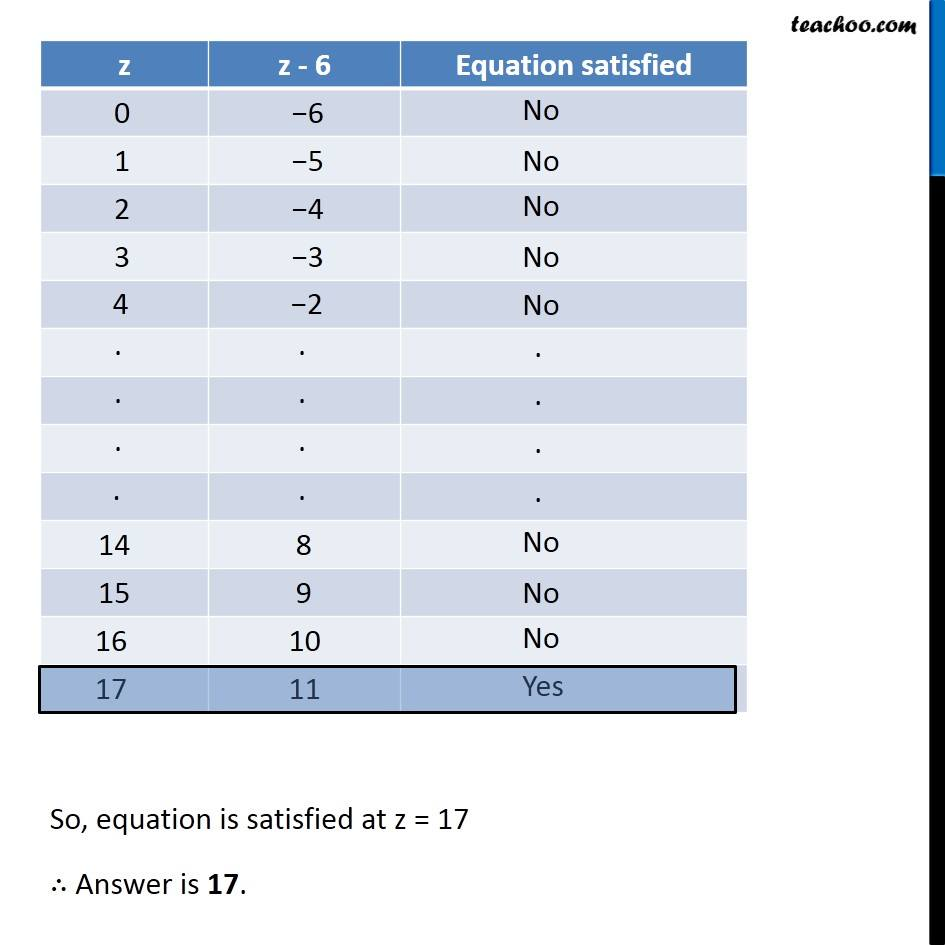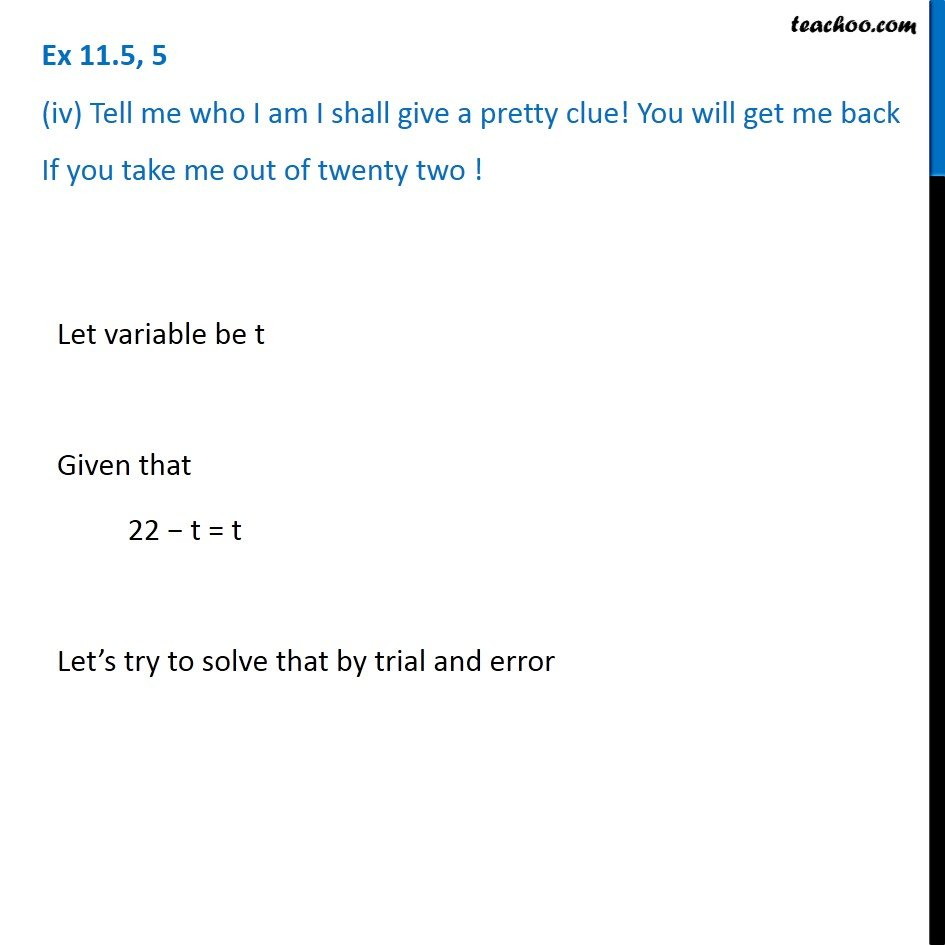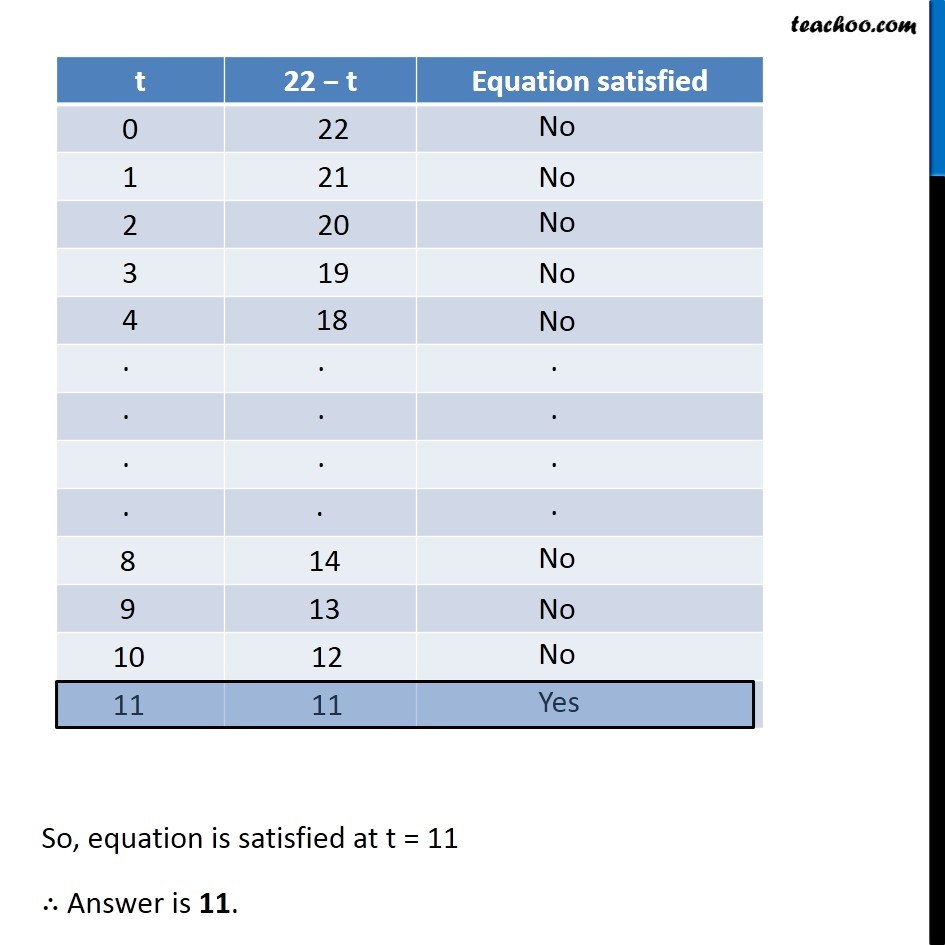Subscribe to our Youtube Channel - https://you.tube/teachoo

1. Chapter 11 Class 6 Algebra
2. Concept wise
3. Solution of equations

Transcript

Ex 11.5, 5 Solve the following riddles, you may yourself construct such riddles. Who am I? (i) Go round a square Counting every corner Thrice and no more! Add the count to me To get exactly thirty four !Let variable be x Since square has 4 crores & we go around it 3 times Our count = 12 Given that, Variable + Count = 34 x + 12 = 34 Let’s solve this by trial & error Equation is satisfied at x = 22 ∴ Answer is 22 Equation is satisfied at x = 22 ∴ Answer is 22 Let the variable be y Since, a week has 7 days Our count = 7 Given that, Variable + Count = 23 y + 7 = 23 Let’s solve this by trial & error So, equation is satisfied at y = 16 ∴ Answer is 16 Ex 11.5, 5 (iii) I am a special number Take away from me a six! A whole cricket team You will still be able to fix !Let variable be z We know that Cricket team has 11 players. Given that, Variable − 6 = 11 z − 6 = 11 Let’s try to solve that by trial and error So, equation is satisfied at z = 17 ∴ Answer is 17. Ex 11.5, 5 (iv) Tell me who I am I shall give a pretty clue! You will get me back If you take me out of twenty two ! Let variable be t Given that 22 − t = t Let’s try to solve that by trial and error So, equation is satisfied at t = 11 ∴ Answer is 11.

Solution of equations